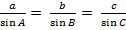Topic Mastery Objectives Essential Knowledge Autumn Term (1st Half term) Perimeter, area and circles · Calculate perimeters and areas of composite shapes made from circles and parts of circles (including semicircles, quarter-circles, combinations of these and also incorporating other polygons); · Calculate arc lengths, angles and areas of sectors of circles; · Give answers in terms of π; · Form equations involving more complex shapes and solve these equations. · Recall and use the formulae for the area of a triangle, rectangle, trapezium and parallelogram using a variety of metric measures 3D forms and volume, cylinders, cones and spheres · Calculate surface area and volume of spheres, pyramids, cones and composite functions · Know the formulae for the volume of a sphere, cone and pyramid · Know the formulae for the surface area of a sphere, a cone and a pyramid Accuracy and bounds · Apply and interpret limits of accuracy, including upper and lower bounds Collecting data · Infer properties of populations or distributions from a sample · Know the limitations of sampling Autumn Term (2ndHalf term) Cumulative frequency, box plots and histograms · Construct and interpret cumulative frequency graphs · Interpret, analyse and compare box plots · Interpret, analyse and compare the distributions of data through measures of spread and quartiles and inter-quartile range · Construct and interpret histograms with equal and unequal class intervals · Know the appropriate use of cumulative frequency graphs · Know appropriate use of box plots · Know appropriate use of histograms · Know interquartile range = upper quartile – lower quartile · Know how to construct a box plot Graphs of trigonometric functions · Sketch or plot and interpret graphs of y = sin x, y = cos x, y = tan x · Know the graphs of the trigonometric functions y = sin x, y = cos x, y = tan x for angles of any size Further trigonometry · Apply the trigonometric ratios in three dimensional figures · Apply the sine rule and cosine rule to find unknown lengths and angles · Apply the sine rule for area to calculate the area, sides or angles of any triangle · Know the sine ruleand the cosine rule a2 = b2 + c2 -2bc cos A · Know area = ½ absinC Quadratics, expanding more than two brackets, sketching graphs, graphs of circles, cubes and quadratics · Expanding brackets of more than two binomials · Deduce turning points of quadratic functions by completing the square · Sketch translations and reflections of a given function · Know the meaning of roots, intercepts and turning points Spring Term (1st Half term) Circle theorems · Apply the standard circle theorems concerning angles, radii, tangents and chords, and use them to prove related results · Know the circle theorems Circle geometry · Apply concepts of rate of change (gradient of chords and tangents) in numerical, algebraic and graphical contexts · Recognise and use the equation of circle with centre origin · Find the equation of a tangent to a circle at a given point Changing the subject of formulae (more complex), rationalising surds, proof · Calculate exactly with surds and rationalise denominators · Re-arrange the subject of a formulae with multiple step or where the subject appears twice · Interpret the reverse process as an inverse function · Interpret the reverse process as the ‘inverse function’ Spring Term (2nd Half Term) Vectors and geometric proof · Apply addition and subtraction of vectors, multiplication of vectors by a scalar and diagrammatic and column representations of vectors · Use vectors to construct geometric arguments and proofs Reciprocal and exponential graphs; Gradient and area under graphs · Plot and interpret graphs of non-standard functions in real life contexts, to find approximate solutions to problems such as simple kinematic problems involving distance, speed and acceleration · Sketch or plot and interpret exponential and reciprocal graphs · Calculate or estimate gradients of graphs are areas under graphs and interpret results in such cases such as distance-time graphs, velocity-time graphs and graphs in financial contexts · Know that graphs of exponential functions y = kx for positive values of k · Know the characteristic shape of the graph of an exponential function · Know the definition of acceleration Direct and inverse proportion · Interpret and construct equations that describe direct and inverse proportion · Understand that X is inversely proportional to Y is equivalent to X is proportional to 1/Y · Know how to set up an equation involving direct or inverse proportion · Recognise graphs that illustrate direct and inverse proportion